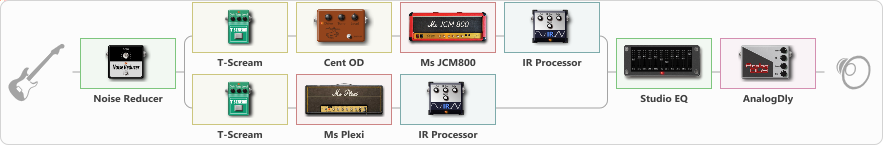# Judas Priest British Steel

Discussion in 'ToneLib-GFX presets' started by Tony the Metalhead, Nov 12, 2021.

1. ### Tony the MetalheadActive Member

Judas Priest British Steel

Preset name: Judas Priest British Steel

Effects chain:Effect: "Noise Reducer" (Dynamics / Filter), active - "yes"
{
"Sens" = 75
"Mode" = Hard
}

Effect: "Splitter" (Dynamics / Filter)
{
"A-Bypass" = Off
"A-Pan" = 0
"A-Level" = 55
"B-Bypass" = Off
"B-Pan" = 0
"B-Level" = 55

'A' branch:
{

Effect: "T-Scream" (Overdrive / Distortion), active - "yes"
{
"Drive" = 80
"Tone" = 40
"Level" = 25
}

Effect: "Cent OD" (Overdrive / Distortion), active - "yes"
{
"Drive" = 65
"Tone" = 55
"Level" = 30
}

Effect: "Ms JCM800" (Amp simulators), active - "yes"
{
"Gain" = 80
"Bass" = 30
"Middle" = 50
"Treble" = 65
"Presence" = 75
"Master" = 75
"Level (dB)" = -7
}

Effect: "IR Processor" (Cabinets), active - "yes"
{
"IR" = OD-R112-V30-E906-P10-20
"Low Cut (Hz)" = 0
"Hi Cut (kHz)" = 20.0
"Mix" = 100
"Level (dB)" = 0
}
}
'B' branch:
{

Effect: "T-Scream" (Overdrive / Distortion), active - "yes"
{
"Drive" = 85
"Tone" = 85
"Level" = 25
}

Effect: "Ms Plexi" (Amp simulators), active - "yes"
{
"Gain" = 75
"Bass" = 30
"Middle" = 40
"Treble" = 55
"Presence" = 85
"Master" = 65
"Level (dB)" = -6
}

Effect: "IR Processor" (Cabinets), active - "yes"
{
"IR" = OD-R112-V30-E906-P10-20
"Low Cut (Hz)" = 0
"Hi Cut (kHz)" = 20.0
"Mix" = 100
"Level (dB)" = 0
}
}
}

Effect: "Studio EQ" (Dynamics / Filter), active - "yes"
{
"31 Hz" = -8
"62 Hz" = -7
"125 Hz" = -4
"250 Hz" = -6
"500 Hz" = -7
"1 kHz" = -6
"2 kHz" = -3
"4 kHz" = -3
"8 kHz" = -5
"16 kHz" = 10
"Level (dB)" = 3
}

Effect: "AnalogDly" (Delay), active - "yes"
{
"Time" = 25
"Feedback" = 25
"Tone" = 50
"Mix" = 35
}

Note: You will need to download and install the ToneLib-GFX software to use the preset.

#### Attached Files:

• ###### Judas_Priest_British_Steel.tlgfx
File size:
8.7 KB
Views:
2,448
Huddled95 and TimurTimofeev like this.